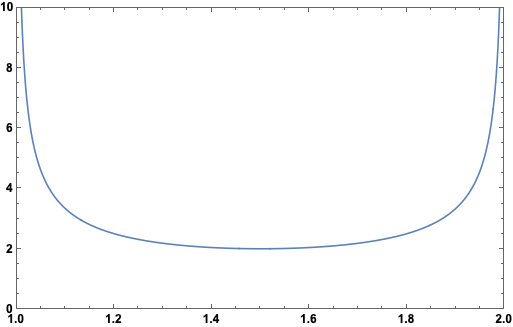# An Interesting Integral

One of my favorite integrals is found in Mechanics by Landau and Lifshitz. In the context of figuring out to what extent the potential energy $$U(x)$$ of a field can be determined if the period of oscillation $$T(E)$$ is known as a function of the energy $$E$$, this integral appears:

$\int_\alpha^\beta \frac{dx}{\sqrt{(\beta - x) (x - \alpha)}} .$

I changed the notation from the book. The assumption is that $$\beta > \alpha > 0$$. With characteristic Russian tersity, the authors write in reference to this integral: “The integral over $$E$$ is elementary; its value is $$\pi$$.”

Here is a plot of the integrand for $$\alpha = 1$$ and $$\beta = 2$$:Mathematica can do this integral if you give it the assumption $$\beta > \alpha > 0$$. But this doesn’t give any insight into why $$\pi$$ is showing up.

One way to solve it by hand is to first complete the square for the term under the square root:

$- x^2 + x (\alpha + \beta) - \alpha \beta = - \left( x - \frac{\alpha + \beta}{2} \right)^2 + \frac{1}{4}(\beta - \alpha)^2 .$

Next let

$u = x - \frac{\alpha + \beta}{2}$

and

$z = \frac{\beta - \alpha}{2} > 0 .$

Making these substitutions, the integral is

$\int_{-z}^z \frac{du}{\sqrt{z^2 - u^2}},$

which is a well-known trig integral:

$\int_{-z}^z \frac{du}{\sqrt{z^2 - u^2}} = \arcsin(1) - \arcsin(-1) = \frac{\pi}{2} - \left( - \frac{\pi}{2} \right) = \pi .$

This solves the integral, but again doesn’t provide much intuition as to why $$\pi$$ shows up. It feels like there should be a nice geometric argument explaining why the integral is $$\pi$$. If you know of one, please let me know!##### Landon Lehman
###### Data Scientist

My research interests include data science, statistics, physics, and applied math.